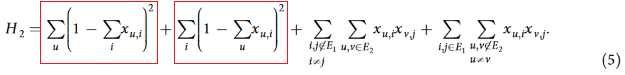# What should be the lowest energy of this graph isomorphism QUBO?

I am trying to study QUBO formulations of the graph isomorphism problem. I tried implementing the QUBO matrices given by the algorithms described in here. For the two input graphs therein, I am getting the matrix  Q given by:

``QUBO_Matrix = `[[-1. 1. 1. 0. 0.] [ 0. -1. 0. 1. 0.] [ 0. 0. -1. 1. 0.] [ 0. 0. 0. -1. 0.] [ 0. 0. 0. 0. -1.]]`

This turns out to be the same matrix as given in the paper. From here, I applied the following lines of code:

``````bqm = dimod.BinaryQuadraticModel.from_numpy_matrix(QUBO_Matrix)
qubo, offset = bqm.to_qubo()

sampler = LeapHybridSampler()
results = sampler.sample_qubo(qubo, label='Graph Isomorphism')

print(results)
______________________________________________
Output:
0  1  2  3  4 energy num_oc.
0  0  1  1  0  1   -3.0       1
['BINARY', 1 rows, 1 samples, 5 variables``````

I think I am doing something fundamentally wrong here as the graphs are isomorphic and as per my understanding, these isomorphic graphs would return a ground state energy of 0 where I am getting a ground state energy of -3.

Even when I am taking non-isomorphic graphs i.e. one of the graphs from the paper and the other, a square graph, I am getting a ground state energy (by the same method) of -1.

I think I am missing something very fundamental here. I would really appreciate it if someone pointed me in the right direction. Thanks in advance!

1 comment
• Hi Turbasu,

Apologies for the delay!

There are a couple of missing details in the paper which might be the reason that the lowest energy for isomorphic graphs is less than zero for your implementation.

In the compact Hamiltonian H_2(equation 5), if you expand the first two terms, you will notice that the linear biases for each x_{u,i} term evaluates to -2. However, in figure 1, Q has diagonal values(representing the linear biases) set to -1. There are no mentions about why or how the biases were scaled down.The second thing to note is that the first two terms also include a constant term which are not included in the implementation. For example, for the given graphs G1 & G2 it will be 1 for each u: [1,2,3] and i: [1,2,4]. So, the offset should be set to 6 and if scaled down by a factor of 2, it evaluates to 3.

I hope looking into these two points helps!

We also have a code example, Circuit Equivalence, which reduces the problem to graph isomorphism. The approach is based on Lucas's formulation as described in the paper "Ising formulations of many NP problems". This example implements the graph isomorphism problem as a discrete quadratic model instead of a BQM and then uses LeapHybridDQMSampler to solve it.